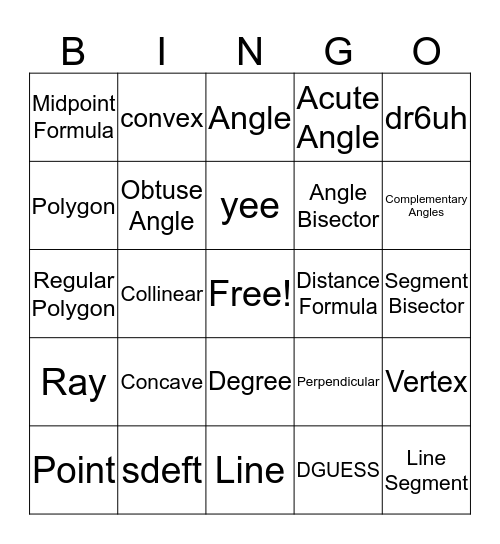# MathThis bingo card has a free space and 24 words: Concave, sdeft, yee, Point, dr6uh, Midpoint Formula, Polygon, convex, Distance Formula, Degree, Angle Bisector, Regular Polygon, Perpendicular, Vertex, Obtuse Angle, DGUESS, Segment Bisector, Angle, Complementary Angles, Collinear, Line Segment, Ray, Acute Angle and Line.

## Play Online